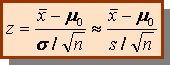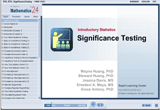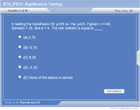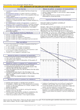How to Learn in 24 Hours?The Rapid Learning Movie

 Need Help? M-F: 9am-5pm(PST): Toll-Free: (877) RAPID-10 US Direct: (714) 692-2900 Int'l: 001-714-692-2900 24/7 Online Technical Support: The Rapid Support Center Secure Online Order:Need Proof? Testimonials by Our Users

 Rapid Learning Courses: MCAT in 24 Hours (2015-16) USMLE in 24 Hours (Boards) Chemistry in 24 Hours Biology in 24 Hours Physics in 24 Hours Mathematics in 24 Hours Psychology in 24 Hours SAT in 24 Hours ACT in 24 Hours AP in 24 Hours CLEP in 24 Hours DAT in 24 Hours (Dental) OAT in 24 Hours (Optometry) PCAT in 24 Hours (Pharmacy) Nursing Entrance Exams Certification in 24 Hours eBook - Survival Kits Audiobooks (MP3)

 Tell-A-Friend: Have friends taking science and math courses too? Tell them about our rapid learning system.Home »  Mathematics »  Introductory Statistics

Significance Testing

 Topic Review on "Title": 5 Steps of Hypothesis Testing Step1: H0: (null hypothesis) Step 2: Ha: (The alternative hypothesis) Step 3: The test statistic and its p-value: Step 4: The critical value and its rejection region Step 5: Conclusion: Reject H0 or Do not reject H0 How do you decide when to reject H0? You need to set a significance level, (e.g., 01 or .05) that represent the maximum risk you can tolerate to have when making a mistake of rejecting H0. Then compare that significance level a with p-value or, compare the statistics with critical value. Hypothesis Test of a Population Mean Suppose We take a random sample n >= 30 from a population with mean m and standard deviation s. s is known (s »  since n is large) We write the hypothesis test as:  Two-tailed: H0:m = m0 Ha: m ¹ m0  One-tailed: Ha: m > m0  or Ha: m < m0 Test statisticsP-value and Critical Value approaches p-value: A p-value is a measure of how much evidence we have against the null hypotheses. The smaller the p-value, the more evidence we have against H0. If p-value< a (significance level), reject H0. P-value and critical approach are equivalent If the p-value <a, reject H0. Conclude that the results are statistically significant at level a. If the p-value > a, do not reject H0. Conclude that the results are not significant at level. If test statistic > critical value, reject H0. If test statistic < critical value, do not reject H0. Two Types of error Define: a = P(Type I error)  = P(reject H0 | H0 is true) b = P(Type II error) = P( do not reject H0 | H0 is false) Our goal is to keep  a, b as small as possible.

Rapid Study Kit for "Title":
 Flash Movie Flash Game Flash Card Core Concept Tutorial Problem Solving Drill Review Cheat Sheet"Title" Tutorial Summary : Significance testing is covered in this tutorial. Detailed description of steps in hypothesis testing is presented along with illustrative examples to guide you through the testing processes to final conclusion. By completing this course, you will learn about the significance testing (hypothesis testing), including introduction to hypothesis testing, two-tailed hypothesis testing, one-tailed hypothesis testing, hypothesis testing for one population mean, difference between two population means, one population proportion and difference between two population proportions

 Tutorial Features: Specific Tutorial Features: Step by step examples showing all details about significance testing are presented. Examples throughout the tutorial facilitate the understanding of many different types of hypothesis testing. Series Features: Concept map showing inter-connections of new concepts in this tutorial and those previously introduced. Definition slides introduce terms as they are needed. Visual representation of concepts Animated examples—worked out step by step A concise summary is given at the conclusion of the tutorial.

 "Title" Topic List: Introduction to hypothesis testing Two-tailed hypothesis testing One-tailed hypothesis testing Hypothesis Testing for One Population Mean Difference Between Two Population Means One Population Proportion Difference Between Two Population Proportions

See all 24 lessons in Introductory Statistics, including concept tutorials, problem drills and cheat sheets:
Teach Yourself Introductory Statistics Visually in 24 Hours